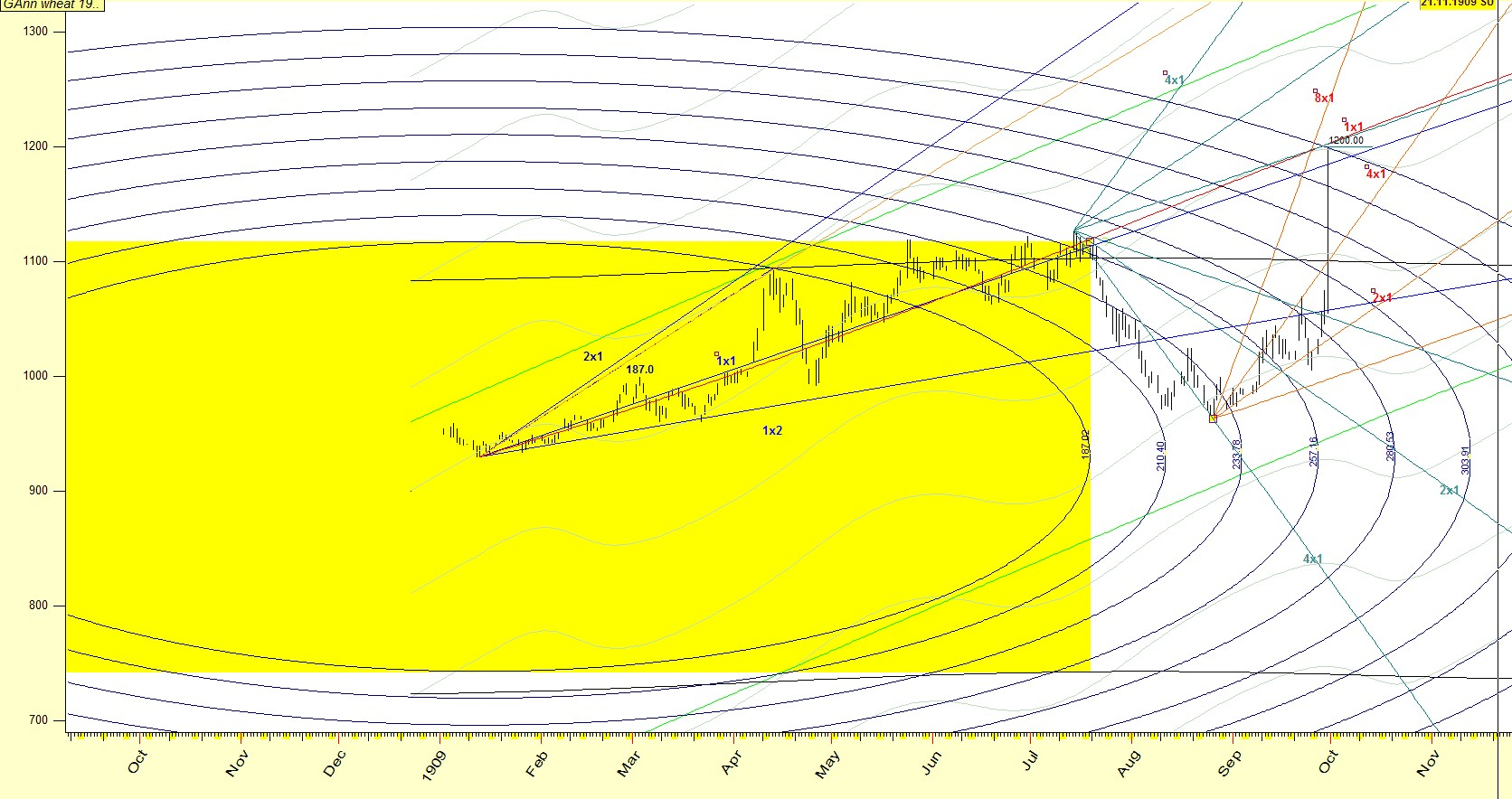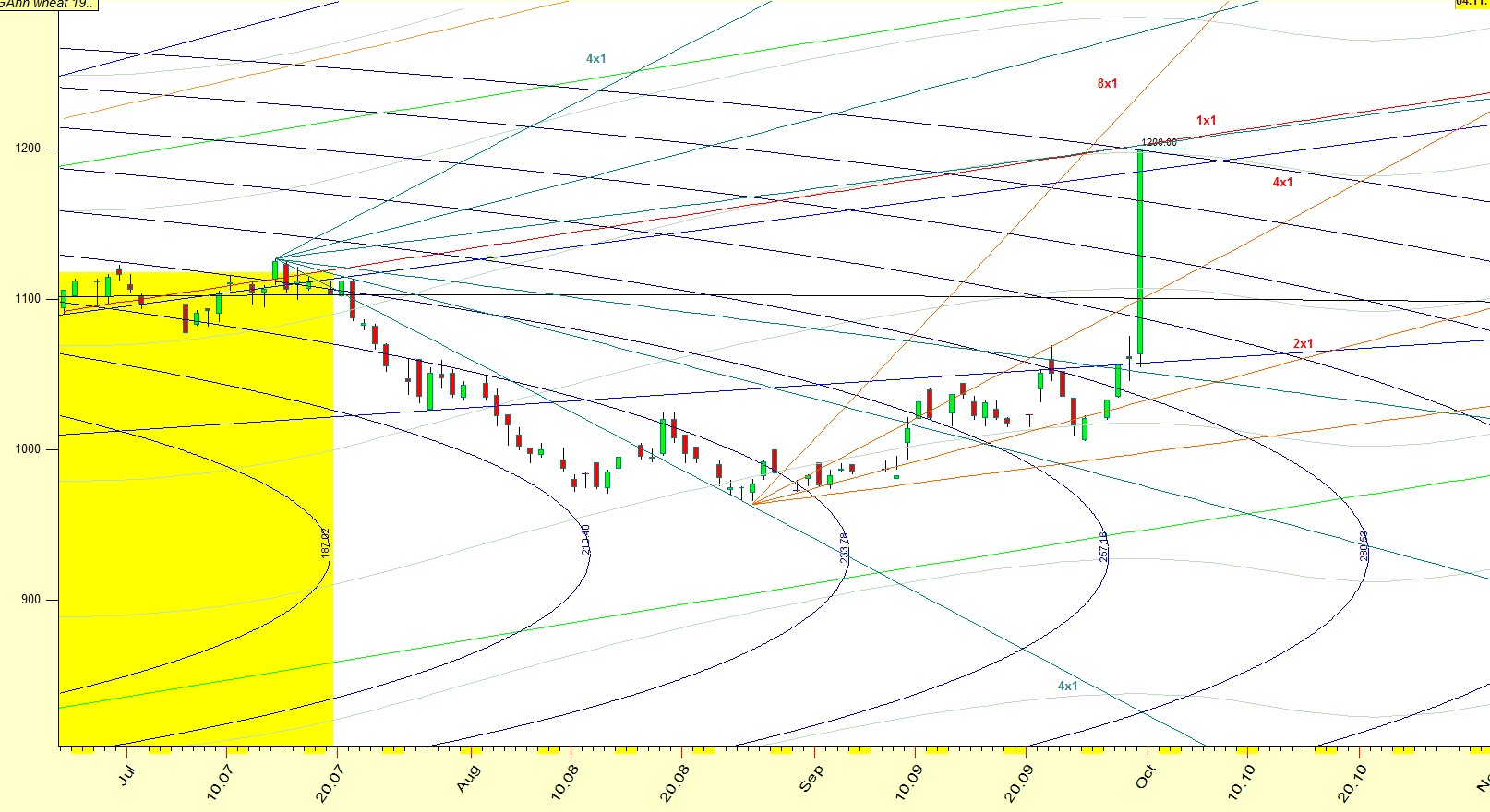## Gann's Wheat Call of September 1909 - analysis 100 years after

Gann insisted on using scaled charts, because he was using his compass to draw vectors from highs to lows and vice versa.

Another reason for which he wanted them properly scaled , was that he layed overlays which had the paths of the planets.

See below:One instance he used all of these techniques was the famous Wheat 1909 call he made.

He said, that if by the end of the trading day of September 29th 1909,Wheat September would not reach 1.20 \$ ,then it would prove that there was something wrong with his method.

Let's see in the chart below what he meant: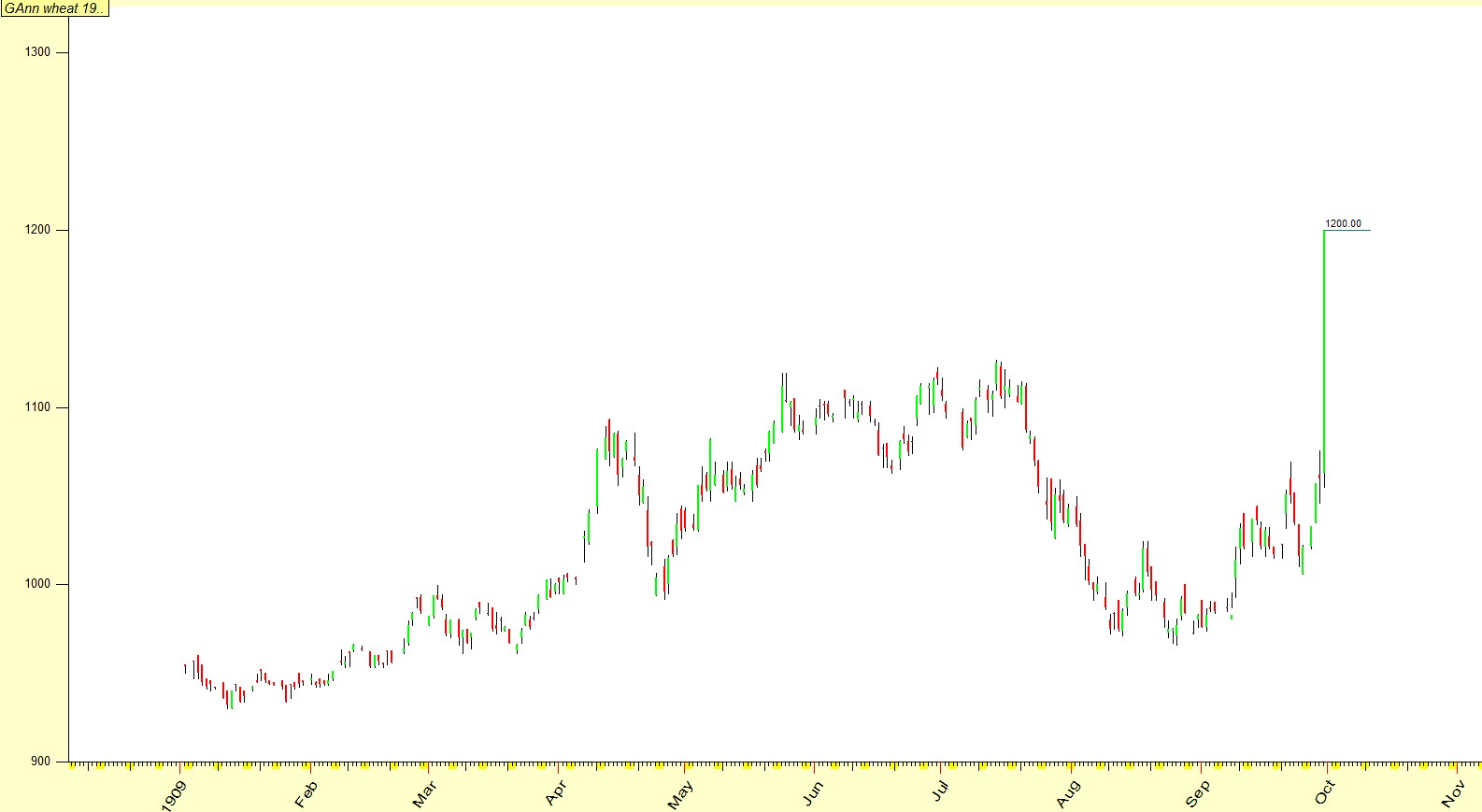This is the chart of wheat September 1909.

January the 13th 1909, wheat made a low price of 930.

As days progressed, Gann was following the mercury planetary lines(gray) and its harmonics (4th).At April 13th 1909, wheat reached a price of 1094.

That was 90 calendar days from the low of January 13th 1909.

A time period which Gann was following in all markets.

He was probably short from then on , as it was a critical day for various reasons.

In many of his books, he would call a price level as a natural level.

That was a name he gave to points in space , where a planetary configurations would occur.

It could be a solar or lunar eclipse, and at other times it could be just a planetary aspect between planets in the geocentric or heliocentric system.

April the 13th was such a date, due to the following planetary configurations:

Mercury and Saturn were conjunct at 14 degrees of Aries, in the Geocentric system

Venus and Saturn were conjunct at 13�30� degrees of Aries, in the Heliocentric system.

Quite a day , as these degrees are not only close, but they convert to the high price of the day.

1094 -360-360-360 or 1094 minus 3 times the circle of 360 degrees,equals 14.

From then on ,as time progressed, Gann was more than sure that these two points, the January low and the high of April 13th were connected in a geometrical way.

He measured the distance from the Low to the High with his compass, and from there he expanded.

What do I mean by that?

Depending on the scale he used for that contract (it could be from 4x4 to 32x32.We don�t know .We do know whether he used 1 point for 1 period or 10 point for 1 period).

In the above diagram, we can see that prices are in the range of 1000s.Thus 1000=1\$

So, measuring with his scaled chart from the price of 930 to the price of 1094 ,he got a radius of :

((1094-930)^ 2 + (90 calendar days) ^ 2) ^.5=187.07 units

Suppose that he charted with 1 inch for r 10 points that would give him a radius of 18.07 inches.

He was fond of 8ths, as these represent the octave.

He would expand his radius with these ratios:

1.125 1.25 1.375 1.5 1.625 1.75 1.875 and finally 2, being one octave.Here you can see the 187 units expanded , by these ratios.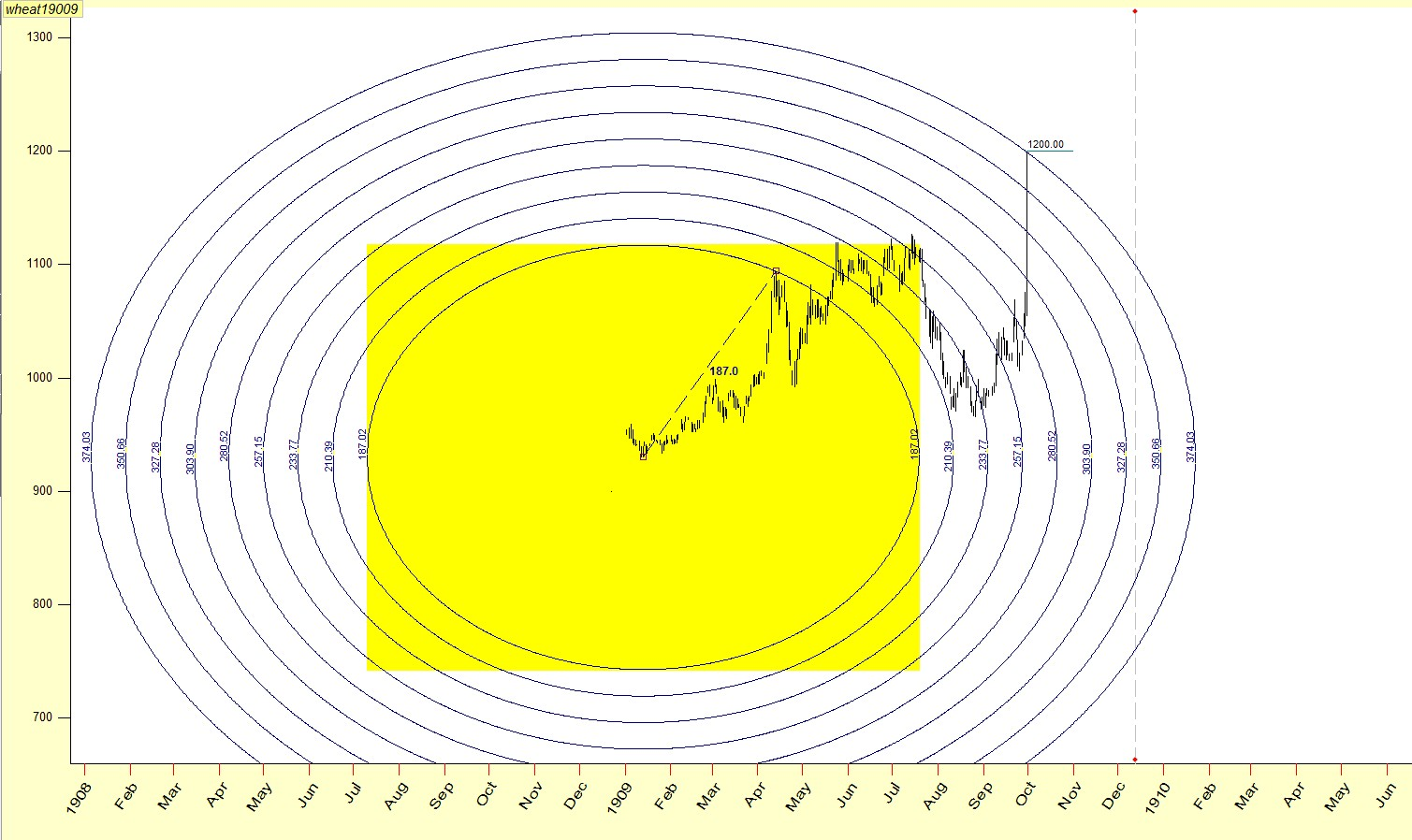On top of that he had his planetary lines:

Black is Saturn=14 degrees Aries (Geocentric)

Green is Venus=14 degrees Aries�(Heliocentric)

Grey is Mercury =14 degrees Aries (Geocentric)You can already see ,the 1200 price being �hit� by mercury and the 200% expansion of the 187.1 vector.

Gann used always his layers on top of his properly scaled charts.

Below, again are the prices ,along with the expansions of the low-high(930-1094) and the 1x2 1x1 and 2x1 angle from the January low(930)

(Set at 1 point per calendar day)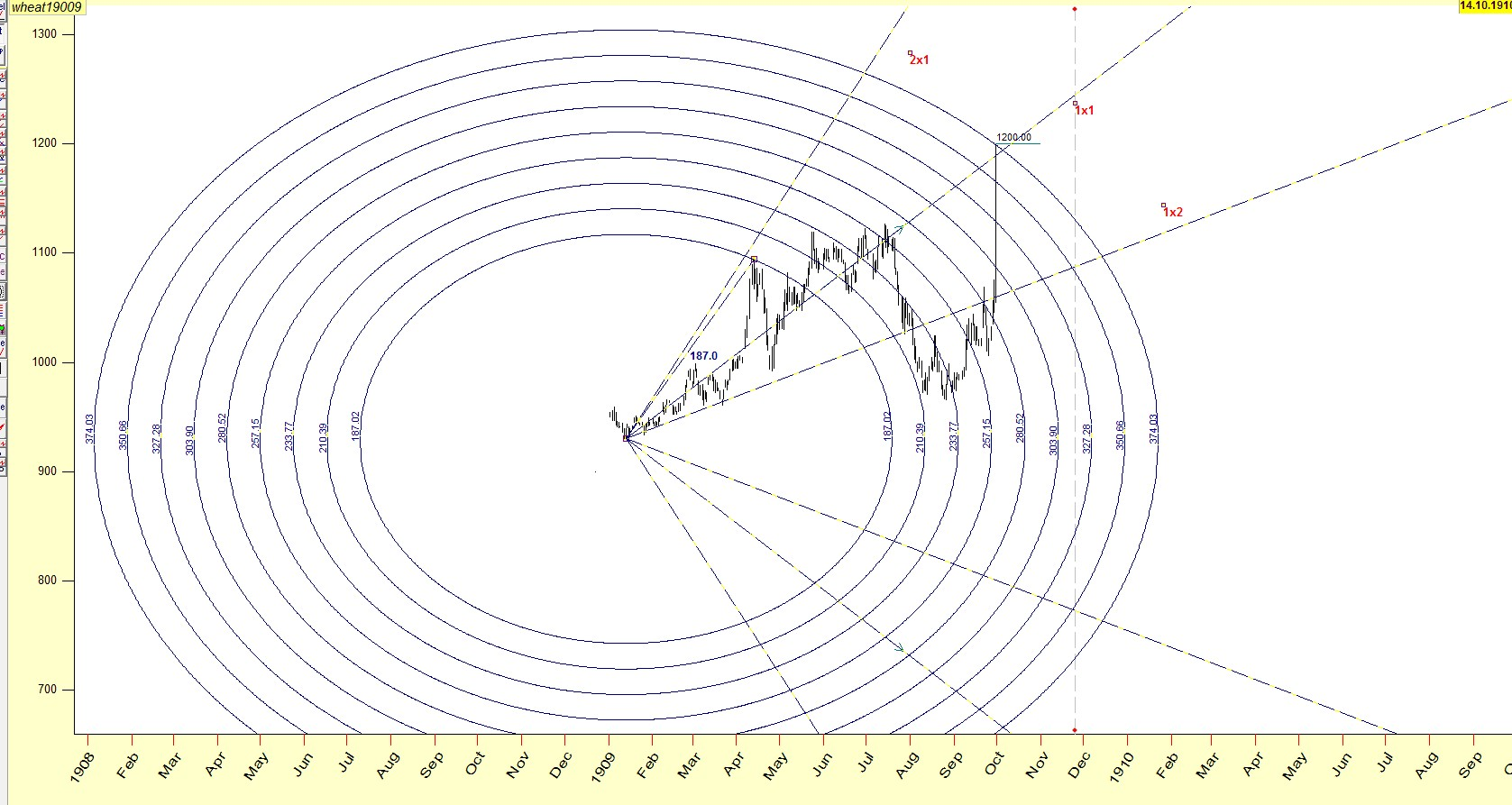Moving forward, we see at Jul 15th 1909,that 183 calendar days had elapsed from the low of 930(January13th 1909)

Or 180 solar degrees.Price reversed at July 14th, 1909 ,and from there Gann as always, used his 1x4 1x2 1x1 2x1 and 4x1 angles from that top,in order to catch the low.

Since 1 point per 1 period in the daily, is close to 1 point per 1 degree of Earth-Sun ,he used that.

See below:

1x1 1 point per 1 degree

2x1 2 point per 1 degree

4x1 4 point per 1 degree

Again ,1200 was hit by the 1x1 from July 14th 1909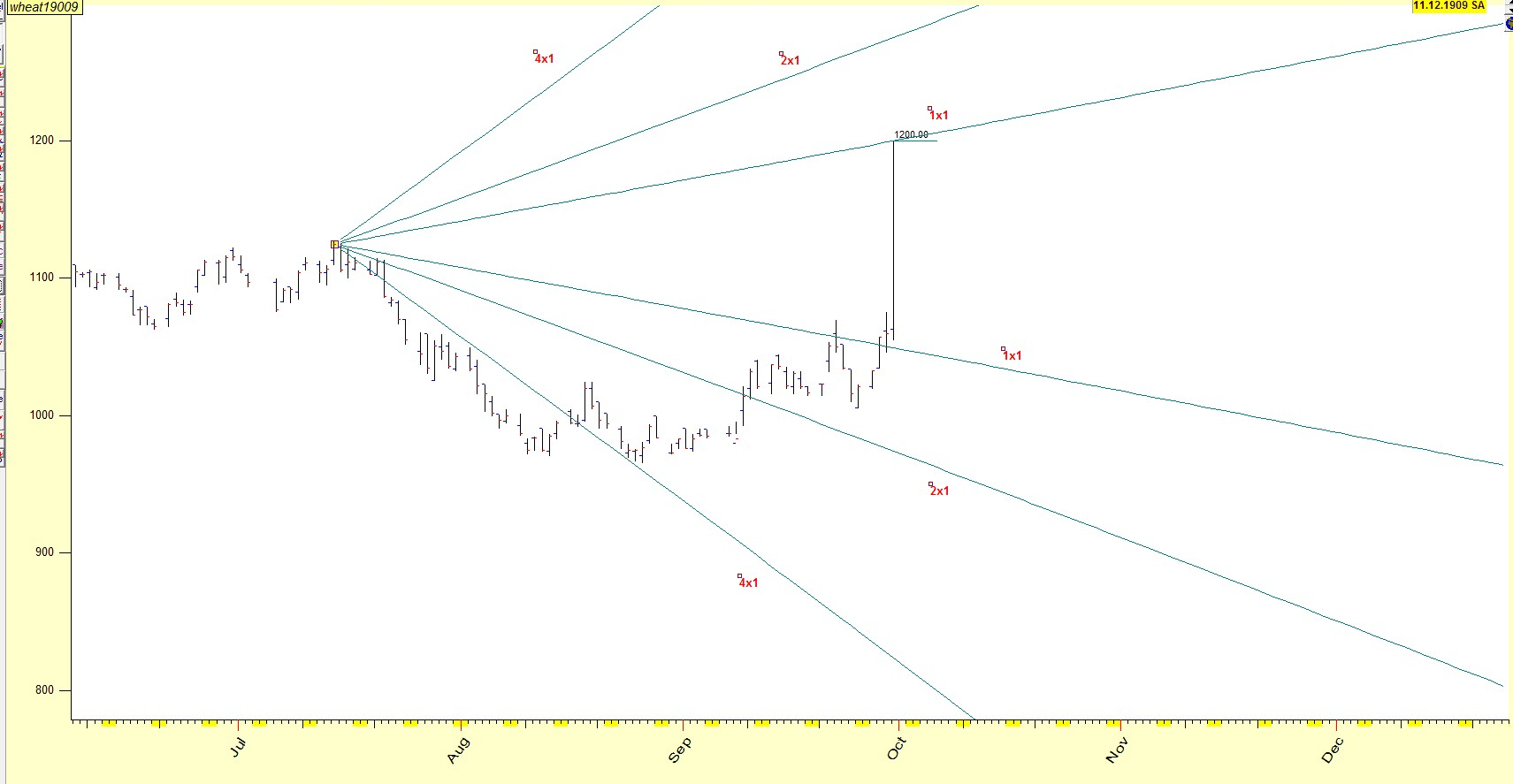The 4x1 SUN angle(4 points per degrees of SUN(earth degrees as SUN doesn�t move))

travelled from the High of July 14th 1909 and caught the price at August 24-25 1909.

From there on he would use again the SUN angles from the low of August 26th 1909 .

See below: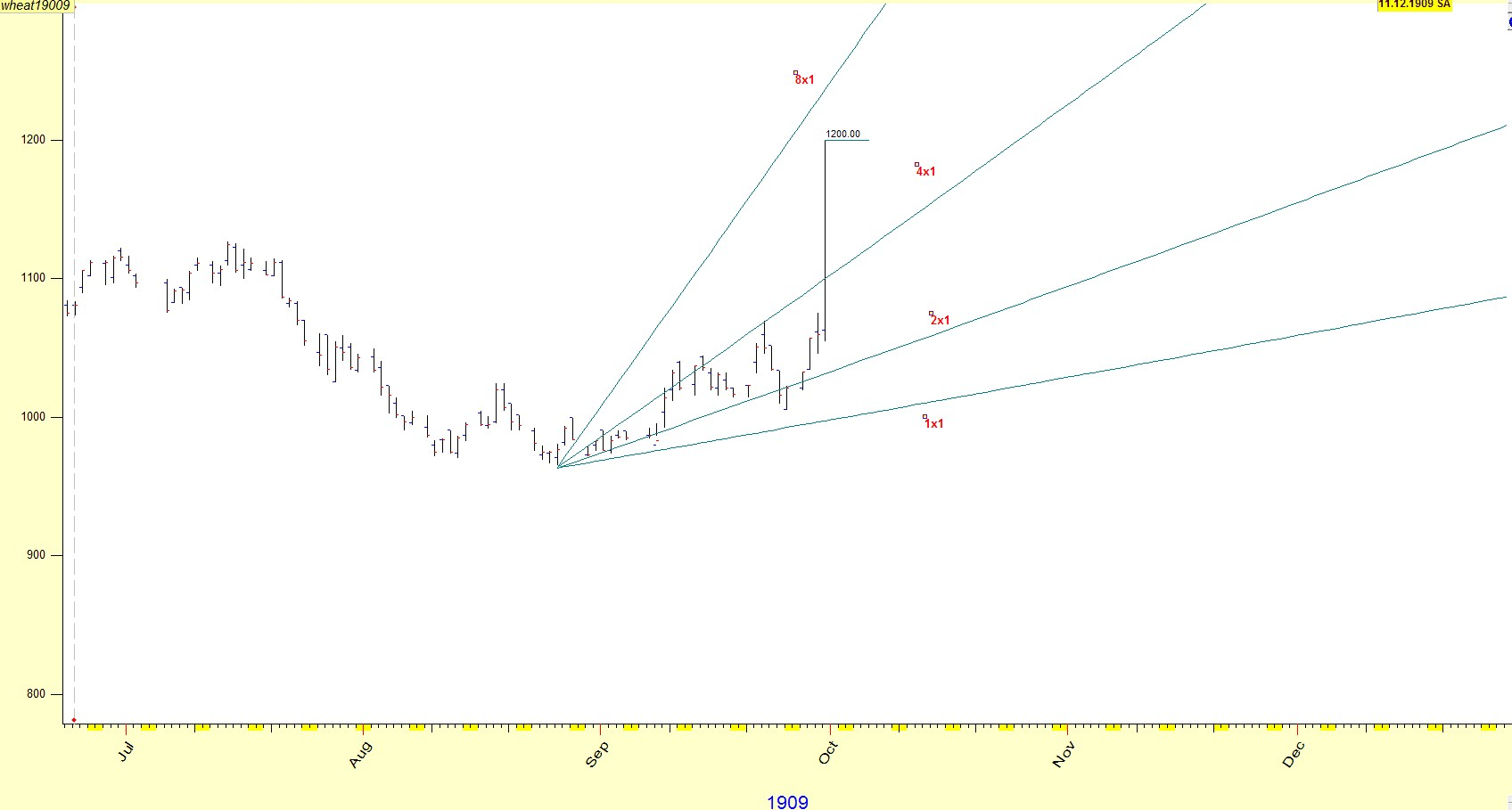These are typical Gann setups.

Lets go back, and see piece by piece what we have so far ,and finally add the �Missing� element wich solves the 1909 Wheat Call.

So far we have seen:

Gann setting up with his compass, vectors.

He have seen the setup once the high of April was reached, due to mercury conjunct Saturn(geocentrically) and Venus conjunct Saturn (heliocentrically).

We have seen SUN angles from the top of July descending, and Sun angles ascending from the low of August

We have seen angles ascending, at 1 point per 1 calendar day ,from the low price of January (930)

I have left the good part for the end.

The setup for Gann�s campaign was set with the mercury planetary lines.

Here they are again:Many of you have noticed that rarely 2 planets set the scene in the market.

It is time to introduce the missing element

Mars

Below you will see what I mean.

The vector of 187(930 to 1094) can be set as a SUN vector

Assigning 1.0569 point per 1 degree of SUN we have the vector of 180(instead of 187) and expanding we arrive at the double (the octave) at 360.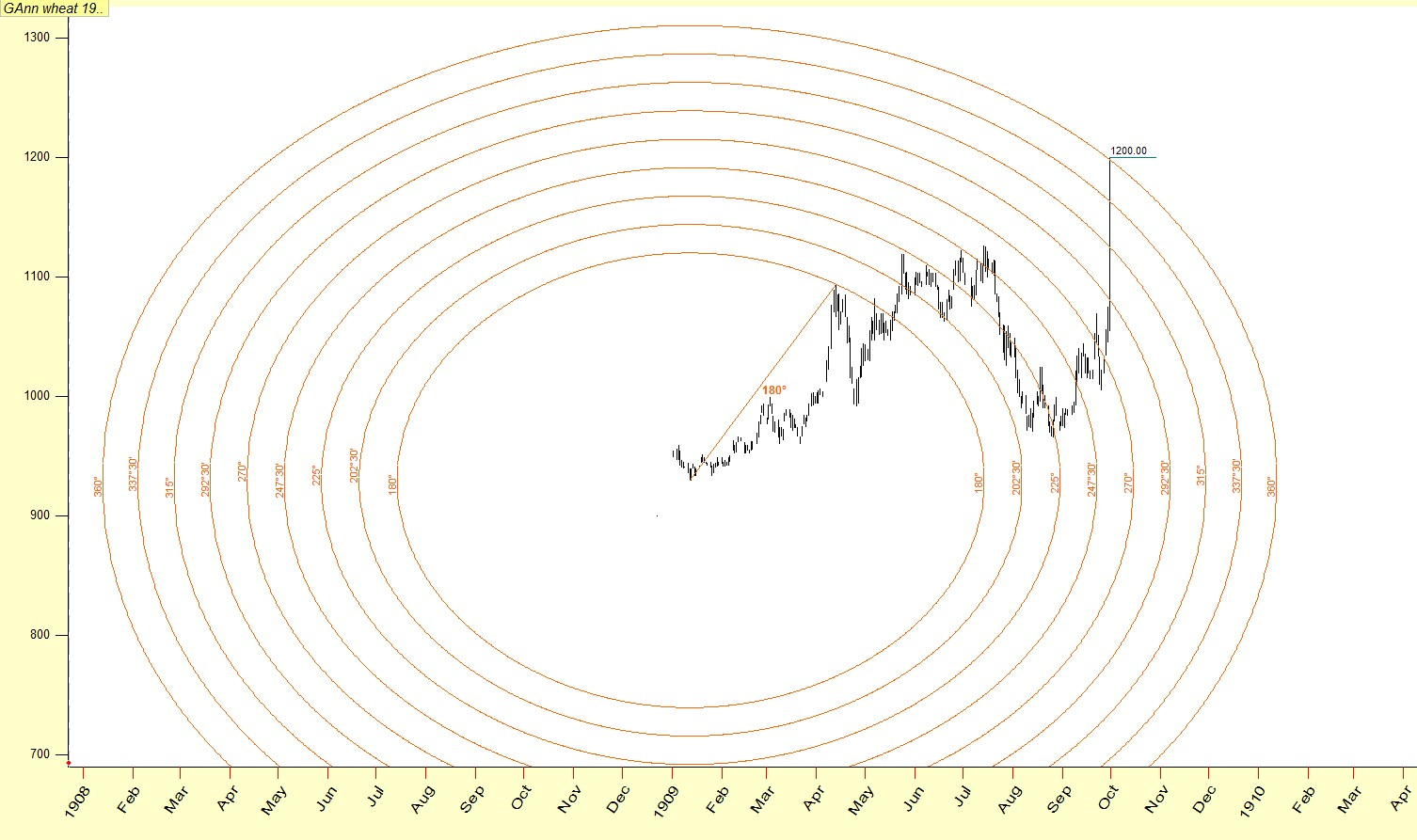Now, assigning the points per Sun degree ,from Low of January 13th 1909 to April 13th 1909,wich is 1.8 point per degree , to a Mars Helio angle from the Low of January 13th,

we get the price of 1200 at September 29th approximately.

See below: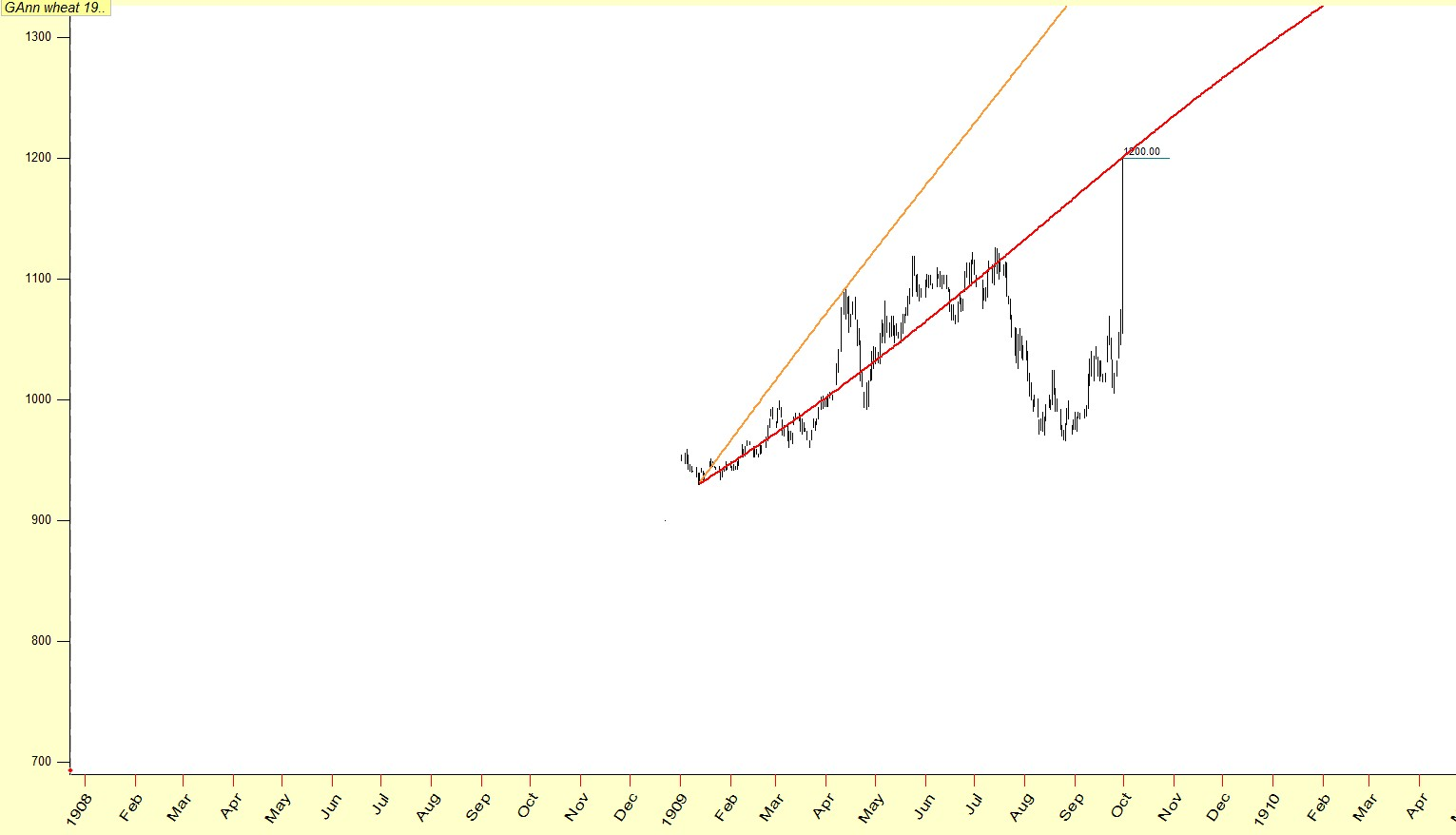The orange line is SUN(or earth) set at 1.8 point per degree ,and the red line is Mars Heliocentic, set again at 1.8 points per degree

We arrive at a price of 1201.5 approximately

This is another confirmation.

See below the whole picture: